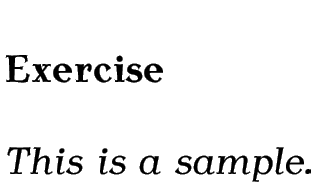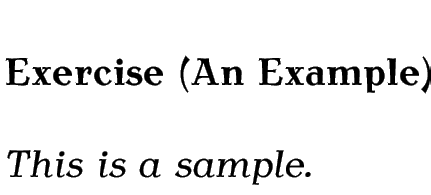Latest news 2023-05-22: new blog post "Bug Bounty Hunters".

# 10. Defining Environments

Just as you can define new commands, you can also define new environments. The command

\newenvironment{<env-name>}[<n-args>][<default>]{<begin-code>}{<end-code>}

is used to define a new environment. As with new commands, you can use the optional argument <n-args> to define an environment with arguments, and <default> to define an environment with an optional argument.

The first argument <env-name> is the name of your new environment. Remember that the environment name must not have a backslash. The mandatory arguments <begin-code> and <end-code> indicate what LaTeX should do at the beginning and end of the environment. Note that although <begin-code> can reference the arguments using #1 etc, the <end-code> part can't.

Example (An Exercise Environment):

Let's first consider an example of an environment without any arguments. Let's make an environment called, say, exercise that prints Exercise in bold and typesets the contents of the environment in italic, with a gap between the title and the contents. In other words, we want the following code:

\begin{exercise}
This is a sample.
\end{exercise}

to produce the following output:(In the next chapter we will add numbering.)

Let's first consider what we want this environment to do: we can get the word “Exercise” in bold using \textbf, and the italic font can be obtained by using the itshape environment (recall §4.5. Fonts). So, at the start of our new environment we need

\textbf{Exercise}\begin{itshape}

and at the end of our new environment we need to end the itshape environment:

\end{itshape}

Putting the above together into the new environment definition:

\newenvironment{exercise}% environment name
{% begin code
\textbf{Exercise}\begin{itshape}%
}
%
{\end{itshape}}% end code

Let's try it out:

\begin{exercise}
This is a sample.
\end{exercise}Not quite right. Let's put a paragraph break after Exercise, and put one before it as well. The command \par can be used to make a paragraph break and the extra bit of vertical spacing can be produced using \vspace. The length \baselineskip is the interline spacing. Modifications are shown in bold like this.

\newenvironment{exercise}% environment name
{% begin code
\par\vspace{\baselineskip}%
\textbf{Exercise}\begin{itshape}%
\par\vspace{\baselineskip}%
}%

{\end{itshape}}% end code

Let's have a look at the output now:[There's a space added after my environment]The indent at the start of each line is caused by the normal paragraph indentation. This can be suppressed using \noindent. It's also a good idea to suppress any spaces immediately following \begin{exercise} and \end{exercise}, which can be done using \ignorespaces and \ignorespacesafterend. Modifications are again shown in bold like this.

\newenvironment{exercise}% environment name
{% begin code
\par\vspace{\baselineskip}\noindent
\textbf{Exercise}\begin{itshape}%
\par\vspace{\baselineskip}\noindent\ignorespaces
}%

{% end code
\end{itshape}\ignorespacesafterend
}

The exercise environment now appears as:Now let's modify our code so that the environment takes an argument. The argument should indicate the exercise topic. For example, the following code:

\begin{exercise}{An Example}
This is a sample.
\end{exercise}


should produce the following result:As with \newcommand, #1 is used to indicate the first argument. We can now modify the code as follows:

\newenvironment{exercise}% environment name
{% begin code
\par\vspace{\baselineskip}\noindent
\textbf{Exercise (#1)}\begin{itshape}%
\par\vspace{\baselineskip}\noindent\ignorespaces
}%

{% end code
\end{itshape}\ignorespacesafterend
}

This book is also available as A4 PDF or 12.8cm x 9.6cm PDF or paperback (ISBN 978-1-909440-00-5).

© 2012 Dickimaw Books. "Dickimaw", "Dickimaw Books" and the Dickimaw parrot logo are trademarks. The Dickimaw parrot was painted by Magdalene Pritchett.